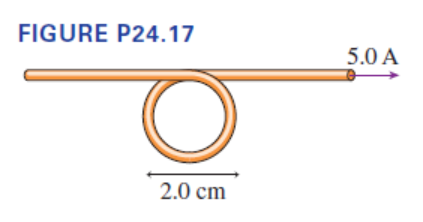# Problem: What is the magnetic field at the center of the loop in Figure P24.17?

###### FREE Expert Solution

The magnetic field will be the sum of the magnetic field at the center of the loop due to thestraight section and due to the circular section.

Magnetic field due to the straight section:

$\overline{){\mathbf{B}}{\mathbf{=}}\frac{{\mathbf{\mu }}_{\mathbf{0}}\mathbf{i}}{\mathbf{2}\mathbf{\pi a}}}$

$\begin{array}{rcl}{\mathbf{B}}_{\mathbf{s}}& \mathbf{=}& \frac{\mathbf{\left(}\mathbf{4}\mathbf{\pi }\mathbf{×}{\mathbf{10}}^{\mathbf{-}\mathbf{7}}\mathbf{\right)}\mathbf{\left(}\mathbf{5}\mathbf{.}\mathbf{0}\mathbf{\right)}}{\mathbf{2}\mathbf{\pi }\mathbf{\left[}\mathbf{\left(}\mathbf{2}\mathbf{.}\mathbf{0}}{\mathbf{2}}\mathbf{\right)}\mathbf{×}{\mathbf{10}}^{\mathbf{-}\mathbf{2}}\mathbf{\right]}}\end{array}$

Bs = 1.0 × 10-4 T

Magnetic field due to the circular section:

84% (72 ratings)###### Problem Details

What is the magnetic field at the center of the loop in Figure P24.17?